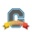# Community

cancel
Showing results for
Did you mean:Community Member

## Is there a way to get the gradebook to weigh all assignments within a grading category equally instead of weighting them according to the number of points possible?

I often make my assignments out of different numbers of points for ease of grading, but a 30-point assignment isn't more important than a 20-point assignment. I want Canvas to average the percentages rather than calculate a group grade according to total number of points. Help?

1 Solution

Accepted SolutionsCommunity Coach

Right now there isn't a way to do this in Canvas, but there are two related feature ideas that have made past the initial voting phase - &

My recommendation is to comment on these ideas and follow them so you'll be alerted to any updates. Until then, if you want Canvas to treat all assignments within an assignment group the same you'll need to make every assignment worth the same number of points. Even if they are worth different points when the students take them, you can standardize them and add the standardized score into Canvas. So if a student got 18/20 (90%) and the standard assignment point value is 30 points then you'd take 30 X .90 = 27 points and then enter in the 27 point into Canvas for that assignment (instead of 18). So no, not perfect, but it would work.Community Coach

Right now there isn't a way to do this in Canvas, but there are two related feature ideas that have made past the initial voting phase - &

My recommendation is to comment on these ideas and follow them so you'll be alerted to any updates. Until then, if you want Canvas to treat all assignments within an assignment group the same you'll need to make every assignment worth the same number of points. Even if they are worth different points when the students take them, you can standardize them and add the standardized score into Canvas. So if a student got 18/20 (90%) and the standard assignment point value is 30 points then you'd take 30 X .90 = 27 points and then enter in the 27 point into Canvas for that assignment (instead of 18). So no, not perfect, but it would work.